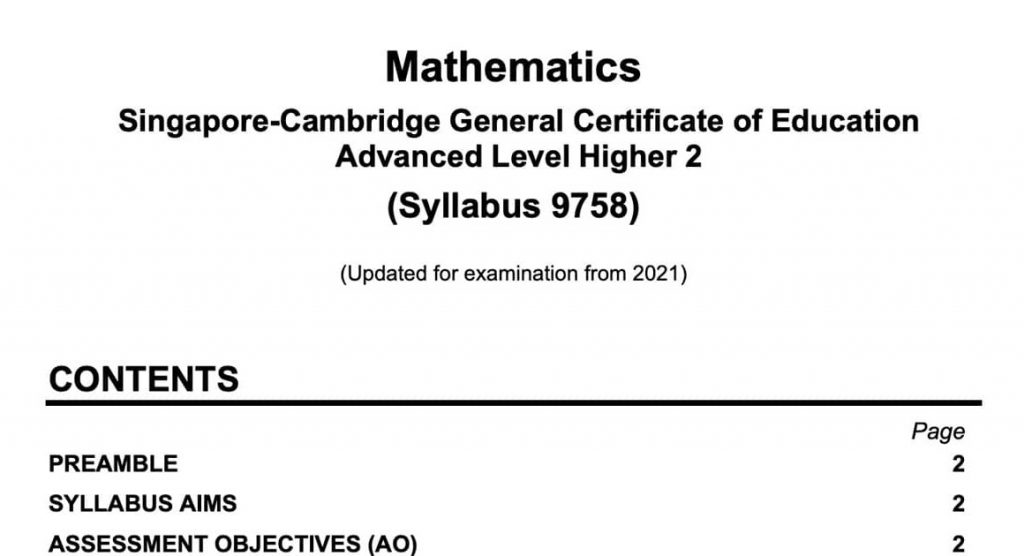# THE MOE 2021 JC H2 MATHEMATICS SYLLABUS EXPLAINED￼

## The MOE 2021 JC H2 Mathematics Syllabus Explained

If you’re going to be sitting for your A-level H2 mathematics examination in 2021, you are probably wondering about the syllabus and the range of topics that would be covered. The syllabus number for your mathematics examination is 9758 and the Singapore Examinations and Assessment Board (SEAB) recently released important information on it.

As the exam is designed specifically to prepare students for various upcoming university courses based on math, it covers a wide range of topics from equations and inequalities to vectors, calculus, statistics and complex numbers.

## The 2021 JC H2 Math Syllabus: An Overview

Anyone who plans to enter the fields of science, mathematics or engineering will need to master the essentials covered in the JC H2 math curriculum.

The full information about Syllabus 9758 is available for viewing here.

Let’s first break down the general topics covered within the syllabus:

• Functions: a broad topic that covers sub-fields like composite functions, inverse functions, conditions for their existence, domain restrictions
• Graphs and transformations: the use of graphic calculation to map out a given graph function, important characteristics of graphs, simple parametric equations and their graphs
• Vectors: basic properties of vectors in two and three dimensions, scalar and vector products in vectors, three-dimensional vector geometry
• Calculus: differentiation, Maclaurin series, integration techniques, definite integrals, differential equations
• Complex numbers: complex numbers expressed in Cartesian form, complex numbers expressed in polar form
• Sequences and series: concepts of sequence and series for finite and infinite cases, sequences as functions, sum and difference of two series, convergence of a series and the sum to infinity, infinite geometric series, etc.
• Statistics and probability: probability, discrete random variables, normal distribution, sampling, hypothesis testing, correlation and linear regression

All of these topics may seem abstract and theoretical when you look at them but SEAB highlights the practical application of each one in higher sciences and university disciplines. Functions and vectors, for example, play a very important role in kinematics and dynamics. Complex numbers and calculus are utilised when making important calculations for electrical circuit establishment, hence electrical engineers need deep knowledge of both fields.

## Exam Parameters

The 2021 JC H2 mathematics exam consists of two three-hour papers for a total test time of six hours. Each of the two carries 50 per cent of the final grade. In addition, each one of the two papers is marked out of 100.

The first paper consists of the following:

• 10 to 12 questions that are based on the pure math sections of the syllabus (functions and graphs, sequences and series, vectors, complex numbers and calculus)
• There will be at least two questions that examine the student’s understanding of how mathematical principles apply to real world problems (usually, within a scientific or engineering context)
• To answer a question, a student may have to draw knowledge from more than one of the pure math fields mentioned above
• Each individual question will carry at least 12 marks
• All questions have to be answered

The second paper consists of the following:

• The second paper has two sections – A and B
• Section A is a pure mathematics exam that adds up to 40 marks. It consists of four to five questions
• Section B is based on probability and statistics and it adds up to 60 marks. Usually, this section is longer and it consists of six to eight questions
• At least two questions in Section B will apply the concepts of probability and statistics to real world problems (usually, within a scientific or an engineering context)
• Students will be expected to answer all questions within the second paper

## Preparing for Your A-Level H2 Mathematics Examination

At this point, you may feel somewhat overwhelmed. The mathematics examination curriculum is very broad and diversified. On top of that, it takes math out of the theoretical realm and establishes a link to real-world applications that most students still do not have first-hand knowledge of.

To top it all off, Syllabus 9758 is also based on some implied knowledge apart from the topics covered. That implied knowledge consists of the content from O-level additional mathematics (algebra, geometry, trigonometry and calculus). As a result, some revision of prior learning material may be required.

The right kind of mathematics tuition is an excellent choice for all students who feel overwhelmed by the syllabus and for those who are looking forward to achieving optimal results.

At the Annexe Project tuition centre, students can benefit from our comprehensive, reliable and understandable A-level H2 mathematics tuition.

Just think about the following accomplishment – in 2019, 92.3 per cent of our students scored either an A or a B in their A-level math exam. Our tuition syllabus is developed to help students master challenging concepts and also sharpen their logical thinking. When these two elements are in place, math problems can be tackled in a highly specific and very efficient manner.

Apart from the general tuition course, we also offer a revision crash course, aimed at helping students quickly overcome challenges and specific problems pertaining to the most complex exam topics.

The A-level H2 mathematics tuition is administered by Annexe Project principal. founder and Math tutor Mr. Alvin Yeo. More information on his competences and qualifications is available here.